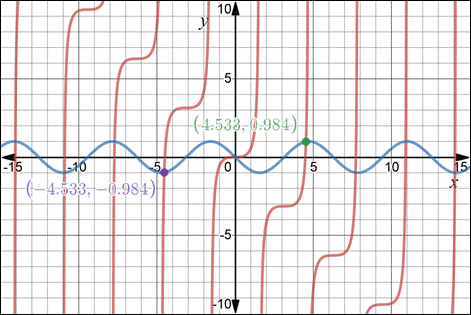# The roots of the curve, y = − sin x .### Single Variable Calculus: Concepts...

4th Edition
James Stewart
Publisher: Cengage Learning
ISBN: 9781337687805### Single Variable Calculus: Concepts...

4th Edition
James Stewart
Publisher: Cengage Learning
ISBN: 9781337687805

#### Solutions

Chapter 4.7, Problem 30E
To determine

## To calculate: The roots of the curve,  y=−sinx .

Expert Solution

The roots are

x14.5

x24.493613

x34.493409 .

### Explanation of Solution

Given information:

The infinitely many lines that are tangent to the curve y=sinx passes through the origin.

Formula used:

Newton’s Method:

We seek a solution of f(x)=0 , starting from an initial estimate x=x1 .

For x=xn , compute the next approximation xn+1 by

xn+1=xnf(xn)f'(xn) and so on.

Equation of tangent line : yy1=m(xx1)

Slope of the tangent line : derivative of the function.

Calculation:

Consider the curve ,

y=sinx

Now,

f(x)=sinxf'(x)=cosx

The line passes through the origin

=yy1=m(xx1)=y0=m(x0)=y=mx (i)

While the tangent will touch the curve at some point. Let the x -coordinate be a.

Therefore , putting x-coordinate in equation y=sinx we get,

y=sina .

The point at which tangent touches the curve is (a,sina) .

Put it in equation (i)

=sina=ma

Slope of the tangent :-

m=cosx .

At point (a,sina) the slope will be

m=cosa

We get ,

=sina=(cosa)a=sinacosa=a=sinacosa=a=tana=a=tanaa=0

Sketching the graph of function y=sinx and y=tanxx . We observe the graph is symmetric.Figure 1.

Hence, we get the slope of the curve is y=tanxx . In other words g(x)=tanxx used to find out roots using Newton’s Method.

Therefore,

g(x)=tanxx and

g'(x)=sec2(x)1

Now, let initial approximation be x1=4.5

For n=1

x2=x1g(x1)g'(x1)

x2=x1tanx1x1sec2(x1)1

x2=(4.5)tan(4.5)4.5[sec(4.5)]21x2=(4.5)4.6373320554.522.50484861

x2=4.50.13733205521.5048486x2=4.50.006386097273x2=4.493613903

The second approximation is x2=4.493613 .

For n=2

x3=x2g(x2)g'(x2)

x3=x2tanx2x2sec2(x2)1

x3=(4.493613)tan(4.493613)4.493613[sec(4.493613)]21x3=(4.493613)4.4977266124.49361321.229544651

x3=4.4936130.00411361220.22954465x3=4.4936130.0002033467422x3=4.493409653

The third approximation is x3=4.493409 .

Hence , the roots of the curve are :-

x14.5

x24.493613

x34.493409

### Have a homework question?

Subscribe to bartleby learn! Ask subject matter experts 30 homework questions each month. Plus, you’ll have access to millions of step-by-step textbook answers!